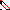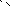# MINLPLib

### A Library of Mixed-Integer and Continuous Nonlinear Programming Instances

#### Instance ex9_2_7

 Formatsⓘ ams gms lp mod nl osil pip py Primal Bounds (infeas ≤ 1e-08)ⓘ 17.00000000 p1 ( gdx sol ) (infeas: 4e-16) Other points (infeas > 1e-08)ⓘ Dual Boundsⓘ 16.99999998 (ANTIGONE)16.99999998 (BARON)17.00000000 (COUENNE)17.00000000 (GUROBI)17.00000000 (LINDO)17.00000000 (SCIP) Referencesⓘ Floudas, C A, Pardalos, Panos M, Adjiman, C S, Esposito, W R, Gumus, Zeynep H, Harding, S T, Klepeis, John L, Meyer, Clifford A, and Schweiger, C A, Handbook of Test Problems in Local and Global Optimization, Kluwer Academic Publishers, 1999.Visweswaran, V, Floudas, C A, Ierapetritou, M G, and Pistikopoulos, E N, A Decomposition-Based Global Optimization Approach for Solving Bilevel Linear and Quadratic Programs. Chapter 10 in Floudas, C A and Pardalos, P M, Eds, State of the Art in Global Optimization, Kluwer Academic Publishers, 1996, 139-162. Sourceⓘ Test Problem ex9.2.7 of Chapter 9 of Floudas e.a. handbook Added to libraryⓘ 31 Jul 2001 Problem typeⓘ QCQP #Variablesⓘ 10 #Binary Variablesⓘ 0 #Integer Variablesⓘ 0 #Nonlinear Variablesⓘ 10 #Nonlinear Binary Variablesⓘ 0 #Nonlinear Integer Variablesⓘ 0 Objective Senseⓘ min Objective typeⓘ quadratic Objective curvatureⓘ convex #Nonzeros in Objectiveⓘ 2 #Nonlinear Nonzeros in Objectiveⓘ 2 #Constraintsⓘ 9 #Linear Constraintsⓘ 5 #Quadratic Constraintsⓘ 4 #Polynomial Constraintsⓘ 0 #Signomial Constraintsⓘ 0 #General Nonlinear Constraintsⓘ 0 Operands in Gen. Nonlin. Functionsⓘ Constraints curvatureⓘ indefinite #Nonzeros in Jacobianⓘ 25 #Nonlinear Nonzeros in Jacobianⓘ 8 #Nonzeros in (Upper-Left) Hessian of Lagrangianⓘ 10 #Nonzeros in Diagonal of Hessian of Lagrangianⓘ 2 #Blocks in Hessian of Lagrangianⓘ 6 Minimal blocksize in Hessian of Lagrangianⓘ 1 Maximal blocksize in Hessian of Lagrangianⓘ 2 Average blocksize in Hessian of Lagrangianⓘ 1.666667 #Semicontinuitiesⓘ 0 #Nonlinear Semicontinuitiesⓘ 0 #SOS type 1ⓘ 0 #SOS type 2ⓘ 0 Minimal coefficientⓘ 5.0000e-01 Maximal coefficientⓘ 5.0000e+00 Infeasibility of initial pointⓘ 7 Sparsity JacobianⓘSparsity Hessian of Lagrangianⓘ```\$offlisting
*
*  Equation counts
*      Total        E        G        L        N        X        C        B
*         10       10        0        0        0        0        0        0
*
*  Variable counts
*                   x        b        i      s1s      s2s       sc       si
*      Total     cont   binary  integer     sos1     sos2    scont     sint
*         11       11        0        0        0        0        0        0
*  FX      0
*
*  Nonzero counts
*      Total    const       NL      DLL
*         28       18       10        0
*
*  Solve m using NLP minimizing objvar;

Variables  objvar,x2,x3,x4,x5,x6,x7,x8,x9,x10,x11;

Positive Variables  x2,x3,x4,x5,x6,x7,x8,x9,x10,x11;

Equations  e1,e2,e3,e4,e5,e6,e7,e8,e9,e10;

e1.. (-5 + x2)*(-5 + x2) + (1 + 2*x3)*(1 + 2*x3) - objvar =E= 0;

e2..  - 3*x2 + x3 + x4 =E= -3;

e3..    x2 - 0.5*x3 + x5 =E= 4;

e4..    x2 + x3 + x6 =E= 7;

e5..  - x3 + x7 =E= 0;

e6.. x4*x8 =E= 0;

e7.. x5*x9 =E= 0;

e8.. x6*x10 =E= 0;

e9.. x7*x11 =E= 0;

e10..  - 1.5*x2 + 2*x3 + x8 - 0.5*x9 + x10 - x11 =E= 2;

* set non-default bounds
x4.up = 20;
x5.up = 20;
x6.up = 20;
x7.up = 20;
x8.up = 20;
x9.up = 20;
x10.up = 20;
x11.up = 20;

Model m / all /;

m.limrow=0; m.limcol=0;
m.tolproj=0.0;

\$if NOT '%gams.u1%' == '' \$include '%gams.u1%'

\$if not set NLP \$set NLP NLP
Solve m using %NLP% minimizing objvar;

```

Last updated: 2023-06-01 Git hash: fdbb0df7